Signup

# Show scores as fractions and percentages

Variables are a great way to track how your respondents answer a quiz or personality test. You can change the value of variables based on respondents’ answers, and then use Logic to show them different Endings, depending on what their variable values are at the end of the typeform.

With Recall information and a bit of math, you can show variable values at the end of the typeform as a fraction (for example, 4/5) or as a percentage (for example, 80%). Read on to learn how!

### Show variable values as fractions

You might show variable values as fractions if you’re building a quiz and want to tell respondents how many total questions they answered correctly.

1. Create a Number variable that tracks correctly-answered questions throughout your typeform. If you haven’t created variables before, read this article to find out how it’s done. You can also use the default score variable.

2. Use Logic to change your variable’s value depending on how questions are answered.

For example, this quiz tests knowledge of world capitals by continent. Questions are grouped by continent, and each continent has a variable. The value of the variable increases by 1 point only when a respondent answers a question correctly: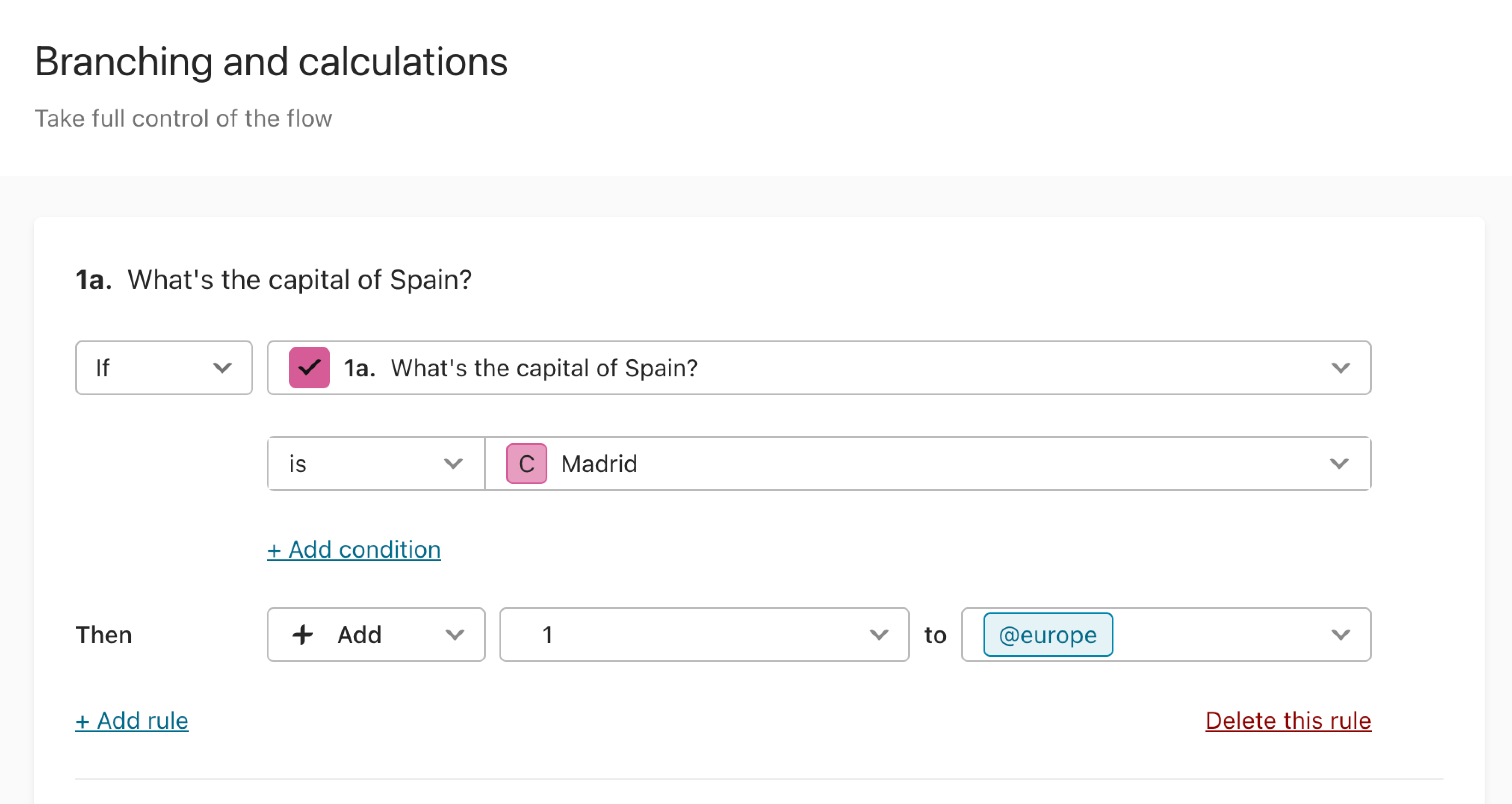3. On your typeform’s Ending screen, use Recall information to show the value of the variable to the respondent. As long as you’ve set up your Logic properly, the variable will show each respondent their own score(s) at the end of the form.

To use Recall information, just click @ to bring up a list of items that you can reference, and choose the variable you want to show: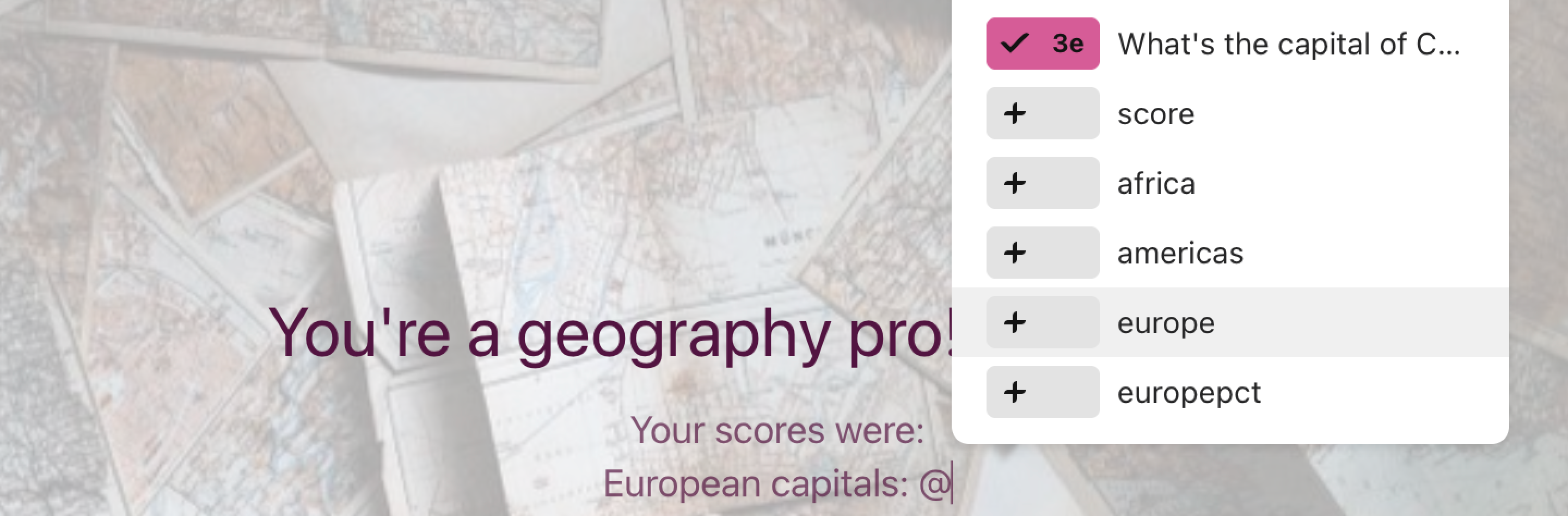Now, show your variable score as a fraction by typing a slash, followed by the total number of questions in your typeform, to the right of the variable. For example, type “/10” if your form has 10 questions. This way, the total number of questions will appear as the fraction’s denominator, while the variable you recalled will be the numerator.

In this quiz’s Ending, for example, there are 3 variables, and each variable has “/5” next to it. That’s because this quiz has 3 sections, with one variable for each section. Each section has 5 questions - 5 questions about European capitals, 5 questions about African capitals, and so on:Someone who answers 3 questions correctly about European capitals, 4 questions correctly about African capitals, and 5 questions correctly about American capitals will see this Ending: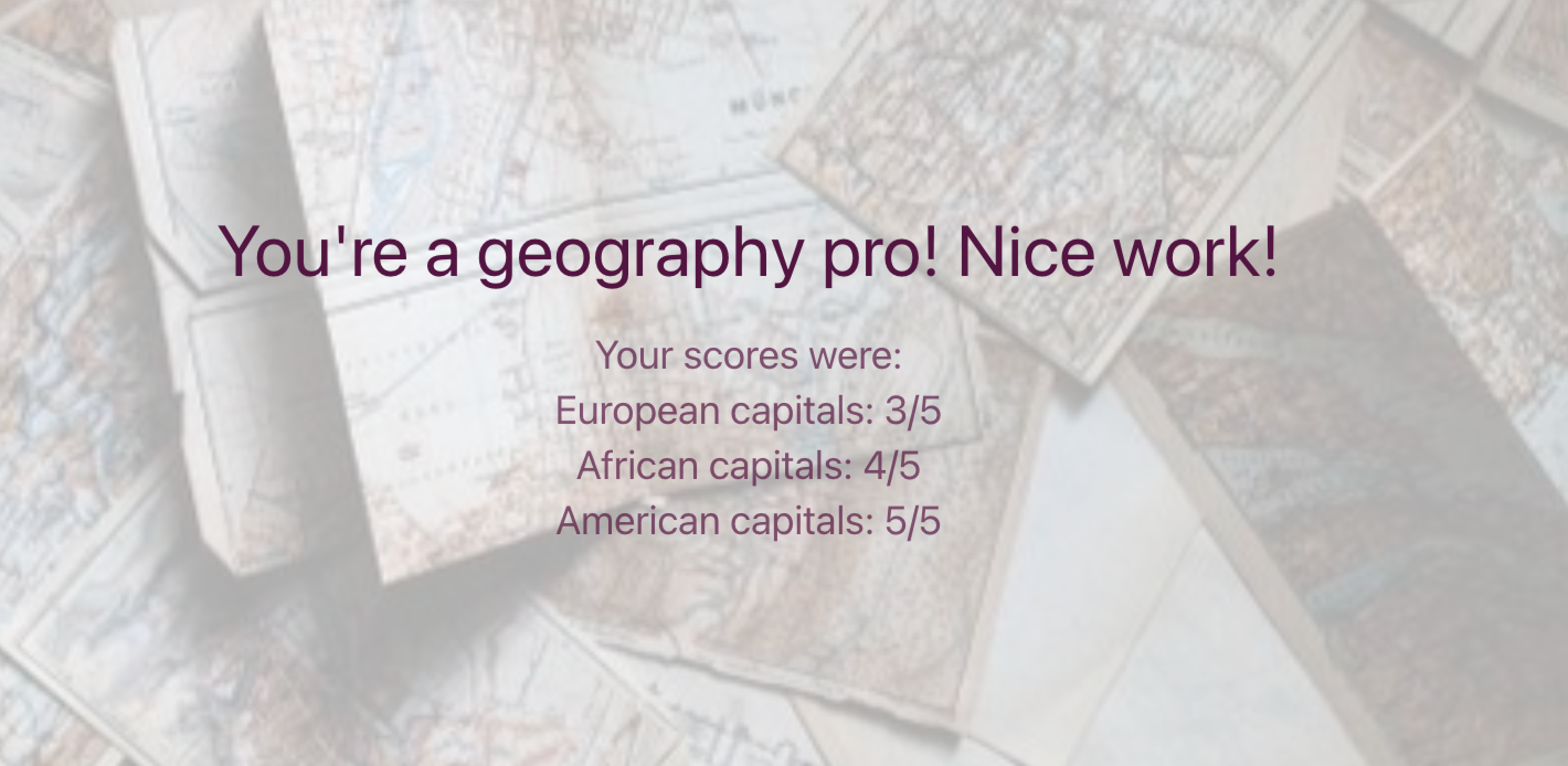### Show variable values as percentages

You might want to show variable values as percentages if you’re building a personality quiz and want to show respondents how much their answers match a particular personality type or trait.

You could also show variable values as percentages if you’re building a knowledge quiz and want to show respondents how well they did overall.

1. Create a Number variable that tracks how questions are answered throughout the typeform. If you haven’t created variables before, read this article to find out how it’s done. You can also use the default score variable.

2. Use Logic to change your variable’s value depending on how questions are answered.

For example, this quiz tests knowledge of world capitals by continent. Questions are grouped by continent, and each continent has a variable. The value of the variable increases by 1 point only when a respondent answers a question correctly:3. Now, add another Logic rule to the last question in your typeform. This rule will convert your variable’s value at the end of the quiz into a percentage-friendly format.

Find the last question in your typeform and click + Add rule: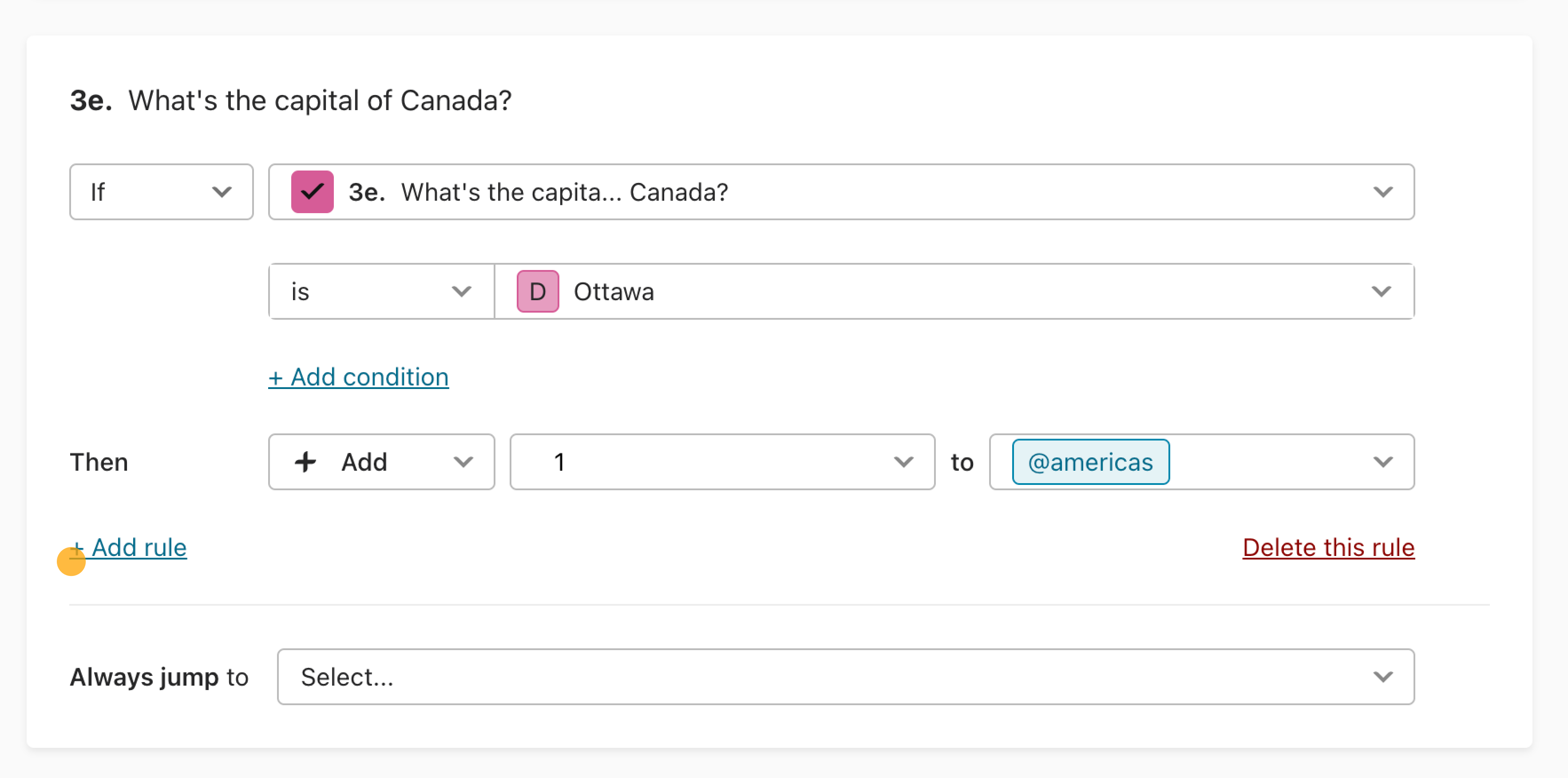Add a rule so that the variable’s value is always divided by the total number of questions in your typeform: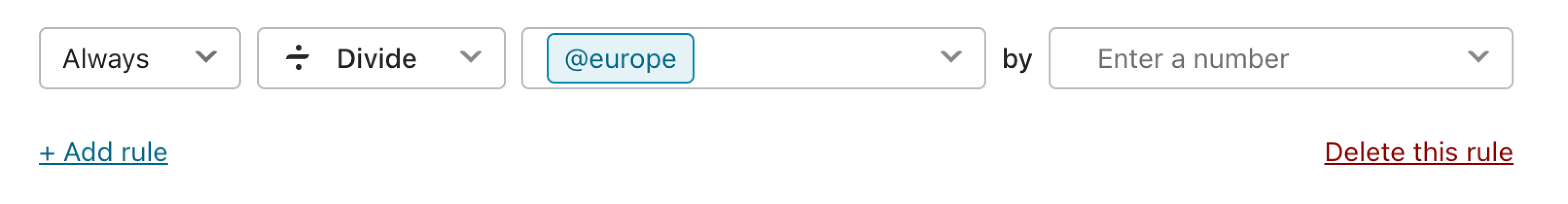This rule creates a decimal that represents the proportion of answers linked to a particular outcome, between 0 (no answers correspond to the outcome) and 1 (all answers correspond to the outcome). In this case, the outcome might be “having a particular personality type” or “getting a perfect score on the quiz”.

Now, click + Add rule again, in the same question.

Add a rule so that the variable’s value is always multiplied by 100:Since percentages are shown as numbers between 0 and 100, this rule will convert your variable’s value into a recognizable percentage format.

Click Save to save your changes.

4. On your typeform’s Ending screen, use Recall information to show the value of the variable to the respondent. If your Logic has been set up properly, the variable will show each respondent their own score, in percentage format, at the end of the form.

To use Recall information, just click @ to bring up a list of items that you can reference, and choose the variable you want to show:Now, type “%” to the right of the variable, to make it clear to the respondent that you’re showing them a percentage score. You can also add additional wording, like “% correct”:Here’s what the Ending screen looks like for the quiz used in this example, which tests knowledge of world capitals. This quiz has 3 sections, each with 5 questions.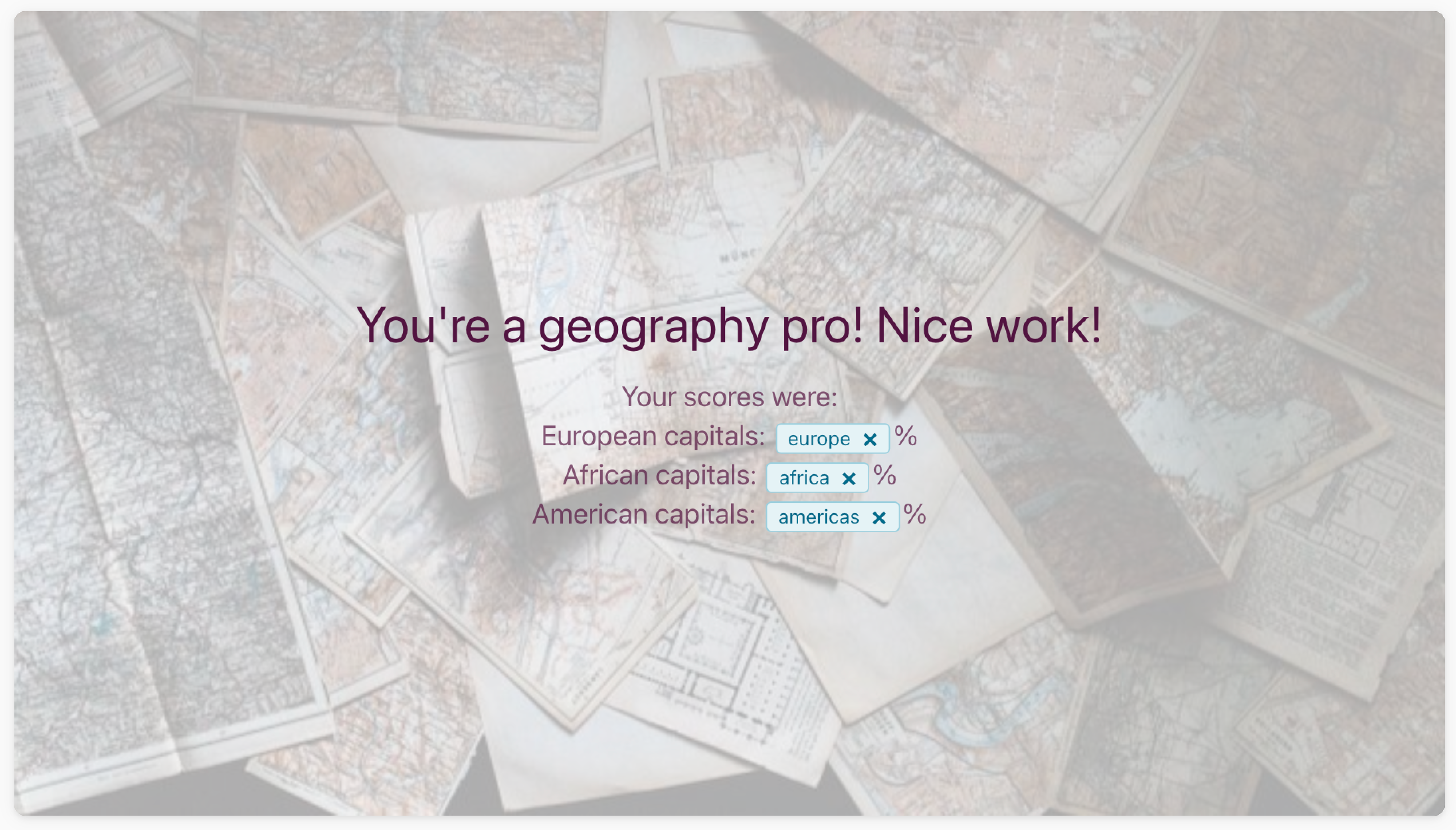Here’s what it looks like after a respondent takes the quiz. In this case, they got 3 questions right in the first section, 4 questions right in the second section, and 5 questions right in the last section: